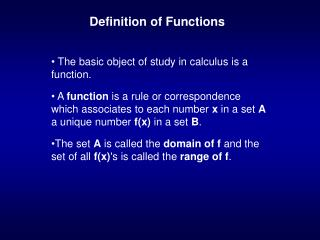Download PresentationDefinition of FunctionsDefinition of Functions - PowerPoint PPT Presentation

Download PresentationDefinition of Functions
An Image/Link below is provided (as is) to download presentation

Download Policy: Content on the Website is provided to you AS IS for your information and personal use and may not be sold / licensed / shared on other websites without getting consent from its author. While downloading, if for some reason you are not able to download a presentation, the publisher may have deleted the file from their server.

- - - - - - - - - - - - - - - - - - - - - - - - - - - E N D - - - - - - - - - - - - - - - - - - - - - - - - - - -
Presentation Transcript

1. Definition of Functions The basic object of study in calculus is a function. A function is a rule or correspondence which associates to each number x in a set A a unique number f(x) in a set B. The set A is called the domain of f and the set of all f(x)'s is called the range of f.

2. Four Representations of a Function • Symbolic or algebraic • Numerical • Graphical • Verbal

3. Continuity If the graph of f breaks at x=x0, so that you have to lift the pencil off the paper before continuing, then f is said to be discontinuous at x=x0. If the graph doesn’t break at x=x0, then f is continuous at x0.

4. Increasing and Decreasing Functions A function is increasing on an interval, if for any a, and b, in the interval f(a) < f(b) whenever a < b. In other words, If the curve of a line is going up from left to right, then it is increasing. A function is decreasing on an interval, if for any a, and b, in the interval f(a) > f(b) whenever a < b

5. One-to-one and Non-one-to-one Functions A one-to-one function maps different inputs to different outputs. In other words, no two x values have the same y value. A non-one-to-one function can map more than one input to the same output.

6. Horizontal Line Test A curve that passes the vertical line test, and thus is the graph of a function, will further be the graph of a one-to-one function if and only if no horizontal line intersects the curve more than once.

7. Basic Functions Constant functions Power functions Inverses of functions Exponential functions Logarithm functions

8. Inverses of Functions Let f be a function with domain D and range R. A function g with domain R and range D is an inverse function for f if, for all x in D, y = f(x) if and only if x = g(y).

9. Exponential Functions Let b be a positive real number. An exponential function with base b is the function: f(x) = bx If the base of the function is e=2.7182818…, a particular irrational number (an infinite non-repeating decimal) between 2.71 and 2.72, the function ex is often referred to as the exponential function.

10. Logarithm Functions Let b be a positive real number. The logarithm with base b f(x) = logb(x) is the inverse of the exponential function g(x) = bx. Informally, we can think of logb(x) as the exponent to which the base b must be raised to give x.

11. Natural Logarithm The natural logarithm f(x) = ln(x) is the inverse of the exponential function g(x) = ex. In other words, ln(x) = loge(x).

12. Laws of Exponents and Logarithms(p. 26) ln ex = x and elnx = x ex ey = ex+y ex/ey = ex-y e -x = 1/ex (ex)y = exy ln ab = ln a + ln b ln (a/b) = ln a – ln b ln (1/a) = - ln a ln (ab) = b ln a

13. Problem - Solving Tactic To solve an equation with the unknown in an exponent, take the logarithm of both sides. To solve an equation with the unknown inside a logarithm, exponentiate both sides.

14. Solving Inequalities Involving Elementary Functions Step 1: Find values of x where f is discontinuous. Step 2: Find the values of x where f is zero. Step 3: Look at the open intervals in between. On each of the intervals, f maintains only one sign.